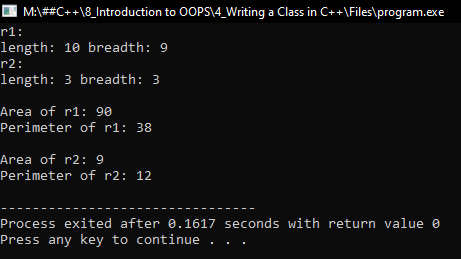# Classes and Objects in C++

## Classes and Objects C++ with Examples:

In this article, I am going to discuss Classes and Objects in C++ with Examples. Please read our previous article where we give a brief introduction to the OOPs concept.

##### What is a class? What is an object?

Most people say that everything in the world is an object and there is a class for it. So let us understand what it means. Classes come from classification. If you take anything in the world you can say that this belongs to so-and-so class, so you can categorize everything. If you take a group of people at a college then you can say that these students belong to computer science, this is a class of computer science and this student belongs to electronics and this is the class of electronics.

So, you’re classifying based on the subject or the course they are going through. It means that classification is based on the criteria that we are adopting. You are grouping a set of students based on their course. So, students are again a class. Students and employees are one classification or categorization, then both students and employees are human beings. So yes, again there is a classification. These are human beings and these are birds and these are animals. So, there are many classes.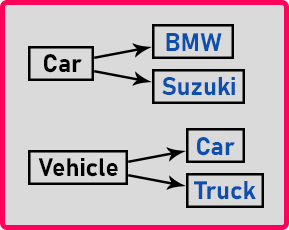Classification is based on some criteria or the common things that you find in them. We define classes in our daily life. Any 4-wheel vehicle in which there is a driver and some people are sitting at the back or beside the driver then we say it’s a car. So, this is the classification depending on the property we are defining that this is a truck or this is a car and both are vehicles again, so the vehicle is again a class.

In this way, if we take examples like human beings, is a class. There’s a class human and you are an object of human being class. The BMW is a car and Toyota is also a car. These are the objects of class cars. So, class is a definition and objects are instances.

Some companies provide housing facilities for their employees. They will have some cargo or apartments or independent houses. All the apartments will be similar only because they follow the same design. So, design is one and there are the houses or the apartments or the flats that are based on the design. This design or the definition is nothing but class and the houses created with the help of that design are objects. Design is nothing but the blueprint of the house object. So, the house will be having all those things that are defined here in the plan.

Now let’s take another example. Suppose an electronics engineer is making a design for a fingerprint scanner and for a fingerprint scanner, he made a design or circuit diagram. Some ICs are there on the circuit. There will be a thumb scanner or fingerprint scanner.

Now there are some fingerprint scanners. All these fingerprint scanners are based on the circuit design. So, the circuit diagram is a class or blueprint of a fingerprint scanner. And all the fingerprint scanners are objects.

So, every engineer does some paperwork or designing work, and based on that design the manufacturing is done. When you make a product, that product will be having all those things that are there in the design and you can then make many products based on that design.

A car company will design a new car. Then they will manufacture many cars based on that design. So, all those cars are objects and the design that the company is holding with them is a class. In the same way, we also want to write a class and create objects in our program.

##### How do we define a class? What will be there in the class?

In the fingerprint scanner example, we used ICs, boards, and pins. And in the house example, there will be walls and pillars and a roof. Then what will be in our classes?

See our product that is software is related to computation. So, we are making classes that are more related to computation. Suppose I want to have a class for Rectangle. Then what it will contain?

We are related to computation which needs data. So, we’ll have only two things, data, and functions. Our class will contain data and functions. Data is called property and function is called behavior.

So, what data is required for Rectangle? It will have length and breadth. So, we will have data that is float length and float breadth. This is about data.

Then what are the function computations we can do? We can find the area of a rectangle and this should give us the float result. And we can find the perimeter of a rectangle and this also gets a float result. We can find the diagonal of a rectangle and this also gets floated results and so on. So, anything in the world, we can define in terms of data and its function.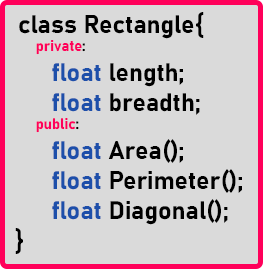This is a class for Rectangle. We have not written a complete class. Now, we will create objects of the Rectangle.

Rectangle r1, r2, r3;

So, these are objects. The object ‘r1’ will have length, breadth, area, perimeter, and so on. And ‘r2’ and ‘r3’ will also have the same things. So, each rectangular object is having everything whatever it is given in this design.

This is how you can write the classes. We can write down the class for anything in the world. So mostly what type of things we deal with in our programming for that we can see it as an object and for that, we can define it as a class, and based on the class we can create an object and use it.

So, in programming, we define this class and keep it, and in our program, let us say the main function and other sets of functions, will be using these objects. So, the question is where to write the class? Shall we write it somewhere else and use it? Yes.

Mostly we write the classes in the same program and there only we will use it. This is our practice, otherwise, you can write some set of classes and keep them in some header files and you can include the header file and used objects of a class.

So that’s all we have given you the difference between class and an object. We have defined a class based on data and functions. And here we create objects and use them.

##### Writing a Class in C++:

Now, we will write a class in C++. We will learn how to write a class and we will learn all the things in detail about the class.

##### How do we define a class, how to create objects and how to use objects?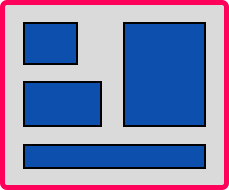As shown in the above image, all the figures are 4-sided figures and these are nothing but rectangles. So, we can say that these are the objects of type rectangle or instances of type rectangle. Each instance is an object of the class Rectangle. We can define a class rectangle in C++.

So, what are the properties or attributes of a rectangle? Every rectangle will have its length and breadth. And what are the operations that we can perform on this rectangle? We can calculate the area; we can find its perimeter and so on. Now let us define a class for the Rectangle.

```class Rectangle{
int length;

int Area(){
}
int Perimeter(){
return 2 * (length + breadth);
}
};
```

Here, length and breadth are the properties or we can say attributes. So, we made them data members. We have taken them of type Integer only. Next, we have defined functions. The Area() function will calculate the area of the Rectangle and it will return the area as an integer type value.

Then we have defined the function for the parameter i.e. Parameter(). It will calculate the perimeter and return an integer type value. After the creation of the class, we have to end it with a semicolon. The semicolon is mandatory. So, this is a class for Rectangle. Now let us see how to use this class. For this, we will write down the main function and use it.

int main(){
rectangle r1, r2;
}

Here we have created objects r1 and r2 of the class Rectangle. We observed two things here. First, we are creating variables. ‘r1’ and ‘r2’ are variables of type Rectangle. See we declare variables of data type as Integer, float, double, or character but here we declare the variables of type Rectangle. Is rectangle a data type? Yes, we have defined our own data type. So ‘Rectangle’ class is used for defining our own data type. So yes, classes are used for defining user-defined data types, so that you can declare the variables of that type. So ‘r1’ and ‘r2’ are Rectangle type. These variables, we call objects.

Now second thing, when we declare any variable inside a function then the variable will occupy memory inside the stack. Yes, we know that. Then ‘r1’ and ‘r2’ variables are also occupying memory inside the stack. How much memory they are occupying? Let us look at the class.

See as part of our discussion we are saying ‘int’ is taking 2 bytes but the compiler that we are using may take 4 bytes. So the ‘Rectangle’ class is having length and breadth, how many bytes they will take? 4 bytes. Functions are also there but functions will not occupy any memory space. So, what is the size of this class? This is 4 bytes. If you create a variable of this class, it will take 4 bytes. So, yes ‘r1’ will take 4 bytes i.e. 2 bytes for length and 2 bytes for breadth, and the same with ‘r2’.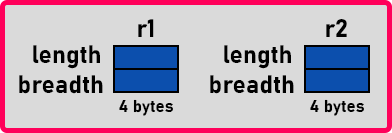Two variables are created inside the stack. Variables will consume memory of four bytes each but the class will not consume. 4 bytes is just the size of a class. It is just like class is the plan of a house. This is a blueprint on paper. Let’s say the house is 100 yards. So, the paper that contains the house design will not occupy a space of 100 yards. But when you construct a house as per the plan it will occupy 100 yards.

So, the creation of an object is over. Now can we use this length and breadth? Yes. We can access data members. For accessing the members of any class, we have to use dot operators. Now one problem, we cannot access this length and breadth. The reason is whatever you write inside the class by default becomes private. Remember this point by default it becomes private. So, we should write everything under public colon.

class Rectangle {
public :

};

After this what all written is public we can access with dot operator. Unless we write public, we cannot access them because by default they are private.

r1.length = 9;
r2.length = 3;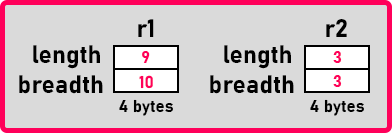Here we have given the values of length and breadth to the objects ‘r1’ and ‘r2’. Now we can calculate area and parameter. So for calculating them we will write,

cout << r1.Area() << endl;
cout << r1.Parameter() << endl;

In the same way, we can write for ‘r2’ also. Both the objects ‘r1’ and ‘r2’ will be having their own area and perimeter function. Also, they will not occupy memory separately for each function. So, there is one class and there are multiple objects and you can create n number of objects.

We define data numbers and functions and we should make everything public when you want to access everything. Then we have created objects of the class and those objects are created inside the stack. And who will occupy memory? Only data members will occupy memory, functions will not occupy any memory but the function works according to the object. So, each object is having its own set of functions.

I have shown you the use of dot operators that are used for accessing data members of an object. We can access data members and also member functions using the dot operator. Now I will show you what it looks like in the Main memory.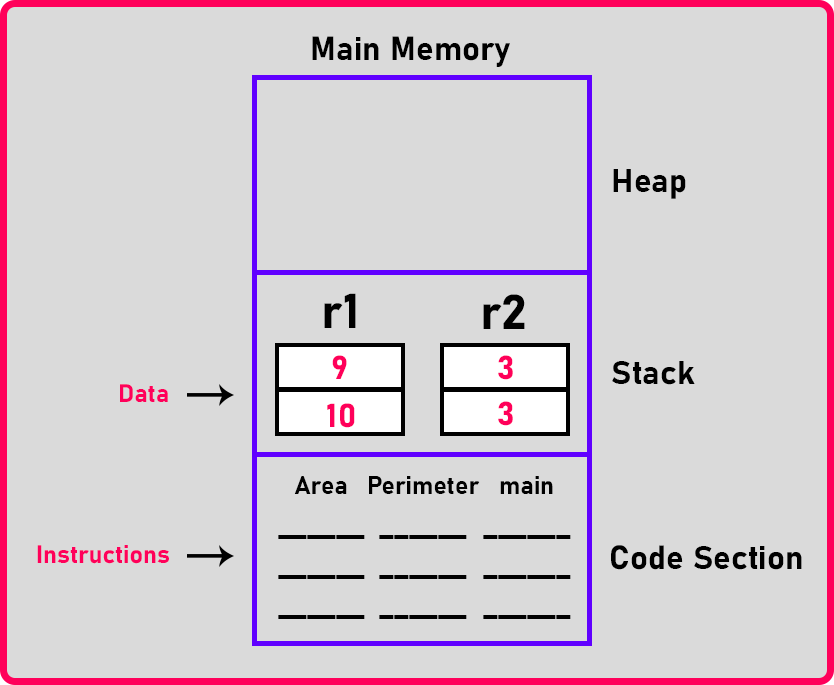In the memory, there are 3 sections that are Heap, Stack, and Code Section. As we are having just the main function so we write it in the code section. Then actually inside the class, we are having two more functions Area() and Perimeter(), then these are also present in the code section.

##### Where is the class?

The class definition is not there, just their functions are present here. Now when the main function starts, two objects are created inside the stack. So ‘r1’ will have its own length and breadth and ‘r2’ will also have its own length and breadth. You can see that a class has two data members and two functions. The data member for each object is separate inside the stack. But for functions, there is only one copy but depending on how you called it like when you said ‘r1.area’ then this function is called upon ‘r1’ and when you said ‘r2.area’ then this function is called upon ‘r2’. That’s it, these are the internal details of a memory.

Now let us write the complete program for the rectangle class.

##### Program showing How to Create Class and Objects in C++:
```#include <iostream>
using namespace std;

class Rectangle
{
public:
int length;

int Area ()
{
}
int Perimeter ()
{
return 2 * (length + breadth);
}
};

int main()
{
Rectangle r1, r2;
r1.length = 10;
r2.length = 3;

cout << "r1:" << endl;
cout << "length: " << r1.length << " breadth: " << r1.breadth << endl;
cout << "r2:" << endl;
cout << "length: " << r2.length << " breadth: " << r2.breadth << endl;

cout << "Area of r1: " << r1.Area () << endl;
cout << "Perimeter of r1: " << r1.Perimeter () << endl << endl;
cout << "Area of r2: " << r2.Area () << endl;
cout << "Perimeter of r2: " << r2.Perimeter () << endl;
}
```
###### Output: